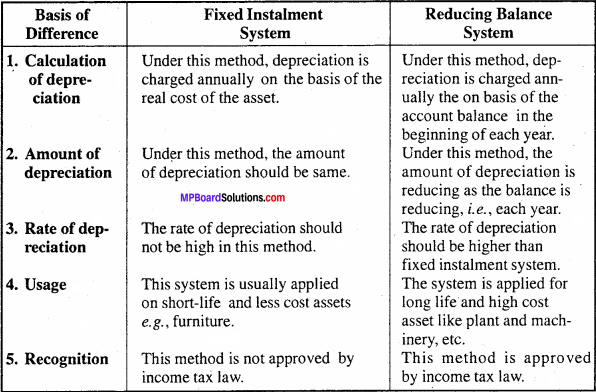## MP Board Class 11th Accountancy Important Questions Chapter 11 Depreciation

### Depreciation Important Questions

Question 1.
What do you mean by depreciation? What is the need of providing Depreciation?
Or
Write objects of providing for depreciation.
Due to constant use of fixed assets in the business, there is continuing diminution or decrease in their value, quantity and quality. This permanent and continuing diminution or decrease in the value of asset is called ‘Depreciation’.

The objects of charging depreciation are as follows:

1. Replacement of fixed assets:
Due to constant use of a fixed asset, it becomes useless after a period of time. Hence, it must be necessary to replace it. At that time the businessman can utilize the money which were written off as depreciation for the replacement of the asset.

2. Proper distribution of loss:
The value of a fixed asset decreases due to its constant use and their loss is relevant to the particular year, i.e., their loss must be accounted each year and that is possible by charging depreciation.

3. For saving tax:
Law provides depreciation as a deduction from income. This reduces the taxable income of the business. So, to save payment of income tax depreciation should be charged.

4. To know accurate profit:
Depreciation is an expense like any other expense. So, only after providing depreciation the real profit for the period can be found.

5. To know the true and fair financial position:
As the value of asset is continually diminishing, it would be very wrong to show them at the original purchase value in the balance sheet. It is only reducing depreciation that the net value of assets can be known.Question 2.
Explain any five reasons for origin of depreciation.
Causes for the Origin of Depreciation : Due to various reasons depreciation arises

1. Continuous use of assets:
In the business concern fixed assets are of daily use, resulting wear and tear, obsolescence and decline in the value of the assets. This decline of value of an asset is technically known as depreciation.

2. Obsolescence:
It means discard of an old machine which becomes uneconomic due to introduction of new machine of latest technique. The latest machine not only ensures cost minimization but the increase in production as well. Thus, for the sake of economy and efficiency of old machine is scrapped despite it being capable of giving service for few more years.

3. Exhaustion of original shape:
Some assets like mines, quarries, etc. exhaust considerably or completely as a result of excavation or removal of resources inherent therein. More and more, excavation / extraction leads to shrinkage or lessening of estimated available quantity. This decline in the value or quantity can be treated as depletion or depreciation.

4. Effluxion or expiration of time:
The value of old machines is always less than that of new machines. This is because, due to the passage of time and charging of depreciation every year the book value of old machine diminishes gradually. After a period of time the value becomes nominal or zero. For example, take leased land of Rs. 50,000 for 15 years. After 10 years its value may be less then half. This decline of value can also be treated as depreciation.

5. Fall in market price:
The market price of the fixed asset may rise or fall. This change in price is of temporary in nature. But the market price of an asset falls due to some special reasons, it can be trated as depreciation.

Question 3.
Write the characteristics of depreciation.
The main characteristics of depreciation are as follows :

1. Depreciation is charged only on fixed assets.
2. Depreciation results in decrease of the value of a fixed asset.
3. By depreciation the value of the fixed asset is constantly declining.
4. Depreciation is a business expense.
5. Depreciation is related with the account of a particular asset only.Question 4.
Write the demerits of reducing balance method.
Demerits of reducing balance method are:

1. No provision for interest on capital – Like fixed instalment method there is no provision of interest on capital invested in the fixed assets.
2. High rate of depreciation – In this method, high rate of depreciation is essential. If the depreciation is charged on low rate, it will take many years to written off the assets.
3. The value of assets can never be zero – This method of depreciation can be applied only when there is some residual value of the asset. In this method, inspite of charging depreciation, the value of assets can never be zero.